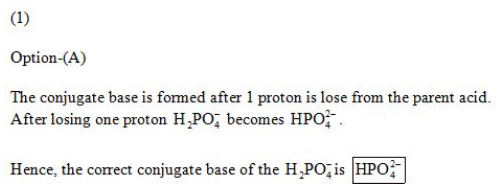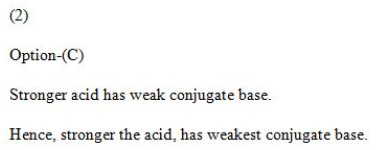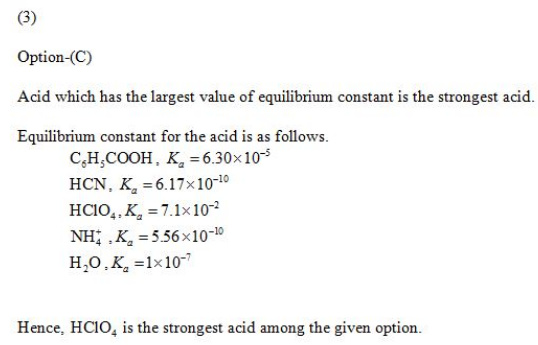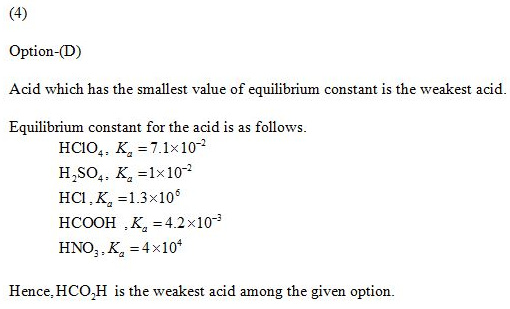# What is the conjugate base of H2PO4⁻? Which of the following is a WEAK acid?

1)What is the conjugate base of H2PO4⁻ ?

A) HPO42-
B) PO43-
C) H3PO4
D) H3O+
E) OH⁻

2)The stronger the acid, then which of the following is true?

A) The stronger the conjugate acid.
B) The stronger the conjugate base.
C) The weaker the conjugate base.
D) The weaker the conjugate acid.
E) None of the above.

3)Which of the following is a STRONG acid?

A) C6H5CO2H
B) HCN
C) HClO4
D) NH4+
E) H2O

4)Which of the following is a WEAK acid?

A) HClO4
B) H2SO4
C) HCl
D) HCO2H
E) HNO3

5)How many of the following are WEAK acids?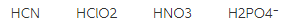A) 0
B) 1
C) 3
D) 4
E) 2

6)Which of the following solutions would have the most basic pH? Assume that they are all 0.10 M in acid at 25∘C. The acid is followed by its Ka value.

A) HF, 3.5 × 10-4
B) HCN, 4.9 × 10-10
C) HNO2, 4.6 × 10-4
D) HCHO2, 1.8 × 10-4
E) HClO2, 1.1 × 10-2

7)Calculate the concentration of OH⁻ in a solution that contains 3.9 x 10-4 M H3O⁺ at 25°C. Identify the solution as acidic, basic or neutral.

A) 2.6 × 10-11 M, acidic
B) 2.6 × 10-11 M, basic
C) 3.9 × 10-4 M, neutral
D) 2.7 × 10-2 M, basic
E) 2.7 × 10-2 M, acidic

8)Calculate the pH of a solution that contains 3.9 x 10-4 M H3O⁺at 25°C.

A) 4.59
B) 3.41
C) 10.59
D) 9.41
E) 0.59

9)Calculate the concentration of H3O⁺ in a solution that contains 5.5 × 10-5 M OH⁻ at 25°C. Identify the solution as acidic, basic or neutral.

A) 1.8 × 10-10 M, basic
B) 1.8 × 10-10 M, acidic
C) 5.5 × 10-10 M, neutral
D) 9.2 × 10-1 M, acidic
E) 9.2 × 10-1 M, basic

10)Calculate the pH of a solution that contains 2.4 × 10-5 M H3O⁺at 25°C.

A) 2.40
B) 9.38
C) 4.62
D) 11.60
E) 4.17

11)Calculate the hydronium ion concentration in an aqueous solution with a pH of 9.85 at 25°C.

A) 7.1 × 10-5 M
B) 4.2 × 10-10 M
C) 8.7 × 10-10 M
D) 6.5 × 10-5 M
E) 1.4 × 10-10 M

12)Determine the pH of a 0.023 M HNO3 solution.

A) 12.36
B) 3.68
C) 1.64
D) 2.30
E) 2.49

13)Determine the pH of a 0.00598 M HClO4 solution.

A) 1.777
B) 6.434
C) 7.566
D) 2.223
E) 3.558

14)Calculate the hydroxide ion concentration in an aqueous solution with a pH of 4.33 at 25°C.

A) 2.1 × 10-10 M
B) 9.7 × 10-10 M
C) 4.7 × 10-5 M
D) 3.8 × 10-5 M
E) 6.3 × 10-6 M

15)Which of the following acids is the STRONGEST? The acid is followed by its Ka value.

A) HF, 3.5 × 10-4
B) HCN, 4.9 × 10-10
C) HNO2, 4.6 × 10-4
D) HCHO2, 1.8 × 10-4
E) HClO2, 1.1 × 10-2